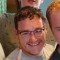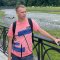# Object ValidationLast updated Mar 3rd, 2021 | Page history | Improve this page | Report an issue

## What is Object Validation in xPDO?¶

Object validation is done through `xPDOValidator`, xPDO's validation class. It's automatically accessible from any `xPDOObject`, via the `getValidator` method.

## How is it Done?¶

Validation can be done either via the XML schema, or during run-time by xPDOValidator methods.

## Example Usage¶

First, let's create our model with this object:

``````<model package="test" baseClass="xPDOObject" platform="mysql" defaultEngine="MyISAM" tablePrefix="test_">
<object class="myTest" table="test" extends="xPDOSimpleObject">
<field key="name" dbtype="varchar" precision="255"
phptype="string" default="" null="false" />

<validation>
<rule field="name"
name="preventBlank"
type="xPDOValidationRule"
rule="xPDOMinLengthValidationRule"
value="1"
/>
</validation>
</object>
</model>
``````

From there, go ahead and generate the model from the XML schema. And now in a Snippet we'll call Test:

``````\$output = '';
\$obj = \$modx->newObject('myTest');
\$validator = \$obj->getValidator();
if (\$validator->validate() == false) {
\$messages = \$validator->getMessages();
foreach (\$messages as \$errorMsg) {
\$output .= 'An error occurred on field "'.\$errorMsg['field'].'": '.\$errorMsg['message'];
}
}
``````

This will output:

An error occurred on field "name": Please specify a name.

## Rules¶

There are three different types of rules, `callable, `preg_match`, and `xPDOValidationRule`.

### The 'callable' Rule¶

A callable rule simply is a rule based upon a function that you pass.

This can be done a few ways. In the "rule" parameter of the schema, you can specify a function name, let's say, 'myCallable', and then make sure to define the function before you call `validate()`.

The function is passed two parameters, the first of which is the value of the column in question, and the second an array of the other attributes on the Rule field in the schema. For example, a model with a rule as such:

``````<rule field="number" name="callable2"
type="callable" rule="myCallable"
min="10" message="Value is too low. Must be 10 or more."
/>
``````

Called with the code:

``````function myCallable(\$value,\$parameters) {
return \$value < \$parameters['min'];
}
\$obj->set('number',101);
\$validator = \$obj->getValidator();
if (\$validator->validate() == false) {
\$messages = \$validator->getMessages();
foreach (\$messages as \$errorMsg) {
\$o .= 'An error occurred on field "'.\$errorMsg['field'].'": '.\$errorMsg['message'].'<br />';
}
}
``````

Will return:

An error occurred on field "number": Callable failed.

You can also call class methods as well; if you have class A with method B, you can make the rule xml attribute be "A::B" to access the function.

### The 'preg_match' Rule¶

A `preg_match` rule is simply a regular expression rule that must pass on a field in order for the object to validate. An example rule in the schema is like such - this one checks to see if the field contains the string 'php':

``````<rule field="name" name="phpMatch"
type="preg_match" rule="/php/i"
message="Does not contain the string 'php'." />
``````

And in the PHP:

``````\$obj->set('name','test');
\$validator = \$obj->getValidator();
if (\$validator->validate() == false) {
\$messages = \$validator->getMessages();
foreach (\$messages as \$errorMsg) {
\$o .= 'An error occurred on field "'.\$errorMsg['field'].'": '.\$errorMsg['message'].'<br />';
}
}
``````

This outputs:

An error occurred on field "name": Does not contain the string 'php'.

### The 'xPDOValidationRule' Rule¶

An xPDOValidationRule rule is a specific rule type that is based upon a class extension of the xPDOValidationRule class. This allows you to do more advanced rules, as well as use the built-in rules. The built in rules include:

More documentation on these specific rules to come.